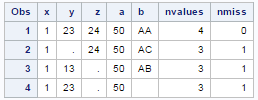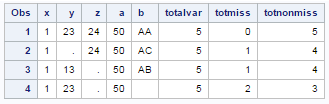# SAS : Count Missing and Non missing Across Columns

This post explains how to count number of missing (blanks) and non missing (non blank) values across multiple columns. It's very easy to calculate it with SAS. It's one of the common data manipulation task that SAS programmers deal in a day to day task but it is mostly associated with finding number of rows or number of non-missing values across rows. There is a rule in SAS that we should remember - We use PROCs when we need to aggregate a COLUMN. Whereas, we use FUNCTIONs to aggregate a row.

Let's create a sample data for demonstration -

The program below creates a sample dataset which would be named as TEMP and it would be stored in WORK library.
data temp;
input x y z a b\$;
cards;
1 23 24 50 AA
1 . 24 50 AC
1 13 . 50 AB
1 23 . 50 .
;
run;
The sample data looks like below -Sample Data

Count Missing and Nonmissing NUMERIC Values

The SAS function N calculates the number of non-blank numeric values across multiple columns. To count the number of missing numeric values, you can use NMISS function.
data outdata;
set temp;
nvalues = N(of x--a);
nmiss = nmiss(of x--a);
proc print;
run;Output
Note - The N(of x--a) is equivalent to N(x, y, z, a). In this case, we are not using the variable b in the above program.

Count total missing and nonmissing values

Suppose you need to calculate number of both character and numeric non-missing and missing values.

Since SAS has no inbuilt function to calculate the number of variables, we need to use PROC CONTENTS to calculate the number of variables. Later we are storing the number of variables information in a macro variable which is totvar.

CMISS Function

The function CMISS counts the number of missing values across columns. It considers missing values of both numeric and character variables.
proc contents data=temp out=cols noprint;
run;

data _null_;
set cols nobs=total;
call symputx('totvar', total);
run;

data outdata;
set temp;
totalvar=&totvar;
totmiss=cmiss(of x--b);
totnonmiss=totalvar- cmiss(of x--b);
proc print ;
run;SAS : OutputShare
Related PostsDeepanshu founded ListenData with a simple objective - Make analytics easy to understand and follow. He has over 10 years of experience in data science. During his tenure, he has worked with global clients in various domains like Banking, Insurance, Private Equity, Telecom and Human Resource.

4 Responses to "SAS : Count Missing and Non missing Across Columns"
1.In the above program, there are 4 observations, and NOBS is used to calculate the number of observations, so how can we calculate number of variables. I mean when we use "set cols nobs=total;" the value of total should be 4 and the value of &totvar should also be 4, but in output it is showing 5. How? I am confused.

1.This comment has been removed by the author.

2.Hey, it is because out statement in proc contents will create a new dataset with the number of variables as number of observation.

3.Hi if I want to list out the names of variables missing by each row how can I do that?

Next → ← Prev
Love this post? Support Us!